# Radical Functions and EquationsPage 1

#### WATCH ALL SLIDES

Slide 1Equations

Slide 2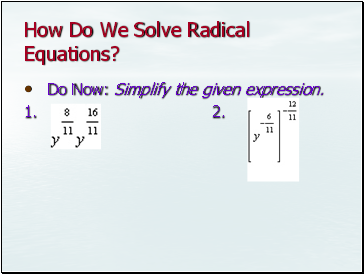## How Do We Solve Radical Equations?

Do Now: Simplify the given expression.

1. 2.

Slide 3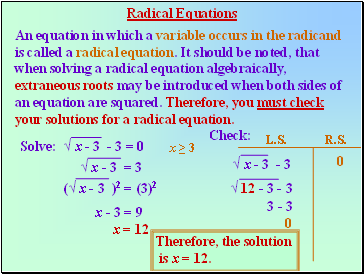An equation in which a variable occurs in the radicand

is called a radical equation. It should be noted, that

when solving a radical equation algebraically,

extraneous roots may be introduced when both sides of

an equation are squared. Therefore, you must check

Solve: √ x - 3 - 3 = 0

√ x - 3 = 3

(√ x - 3 )2 = (3)2

x - 3 = 9

x = 12

Check:

√ x - 3 - 3

√ 12 - 3 - 3

3 - 3

0

0

Therefore, the solution

is x = 12.

x ≥ 3

L.S. R.S.

Slide 4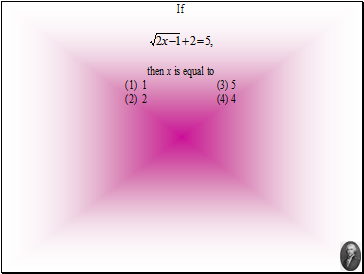Slide 5Slide 6Slide 7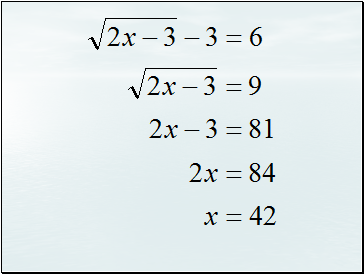Slide 84 + √ 4 + x2 = x

√ 4 + x2 = x - 4

4 + x2 = x2 - 8x + 16

8x = 12

x

Since

the solution of

x =

is extraneous. Therefore,

there are no real roots.

Check:

(√ 4 + x2)2 = (x - 4)2

Slide 9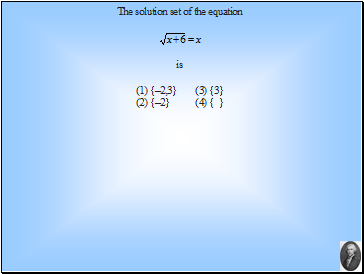Slide 10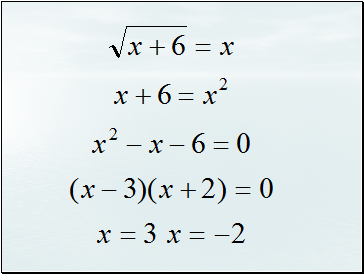Slide 11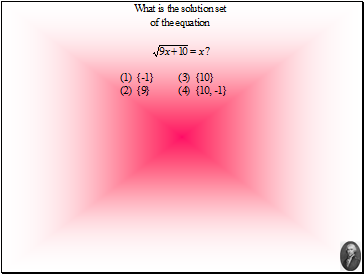Slide 12x = -1 is an extraneous solution.

Slide 13Set up the equation so that

there will be one radical on

each side of the equal sign.

Square both sides.

Simplify.

2x + 4 = x + 7

x = 3

Therefore, the

solution is

x = 3.

x ≥ -2

Solve

L.S. R.S.

Slide 14## Squaring a Binomial

(a + 2)2 = a2 + 4a + 4

Note that the middle term is

twice the product of the two

terms of the binomial.

(a√x + b)2

( 5 + √x - 2 )2

The middle term will

Go to page:
1  2  3